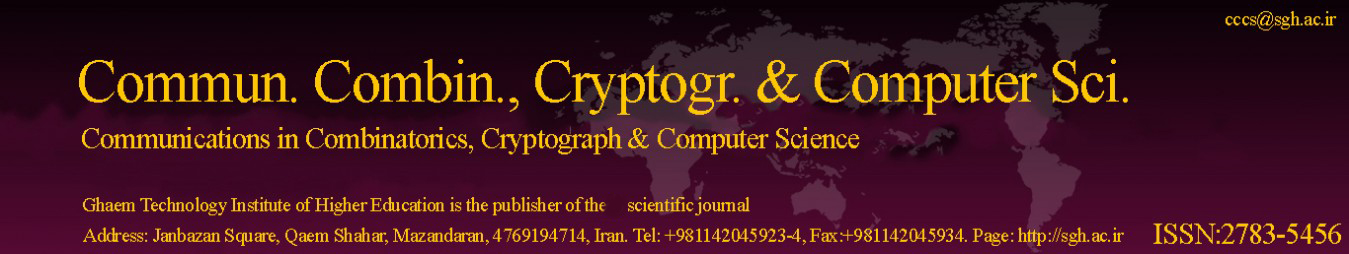Copy the following BibTeX for the article entitled First KCD Matrix and its Laplacian Energy.

BibTeX

@article{Article_24,

title = {First KCD Matrix and its Laplacian Energy},

journal = {Communications in Combinatorics, Cryptography & Computer Science},

volume = {2021},

issue = {2},

issn = { 2783-5456 },

year = {2022},

url = {http://cccs.sgh.ac.ir/Articles/2021/issue 2/2-12-First_KCD_Matrix_and_its_Laplacian_energy.pdf},

author = {Keerthi G. Mirajkar and Akshata Morajkar},

keywords = {First KCD Laplacian matrix, first KCD Laplacian eigenvalues, first KCD Laplacian energy.},

abstract = {This article introduces the concept associated with Laplacian energy for first KCD matrix named as first KCD Laplacian energy. Further, we investigate some basic results related to this concept and also develop significant bounds for first KCD Laplacian energy.}

};